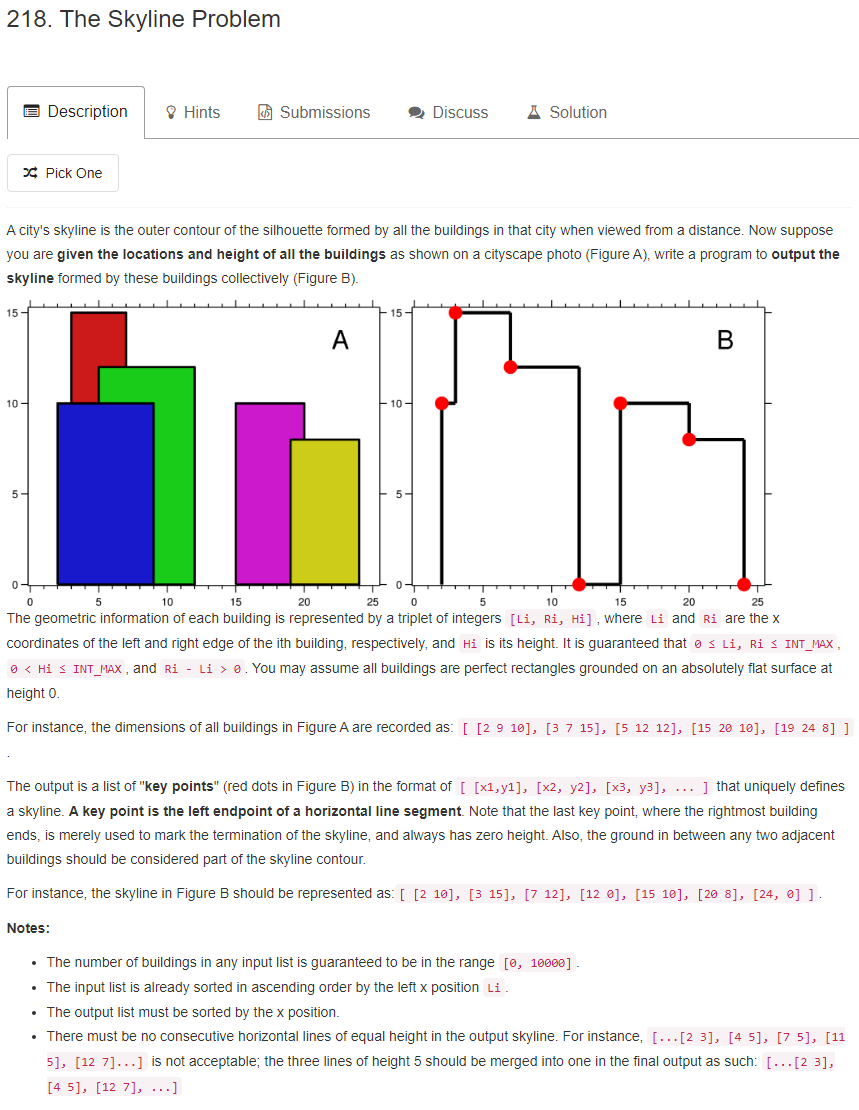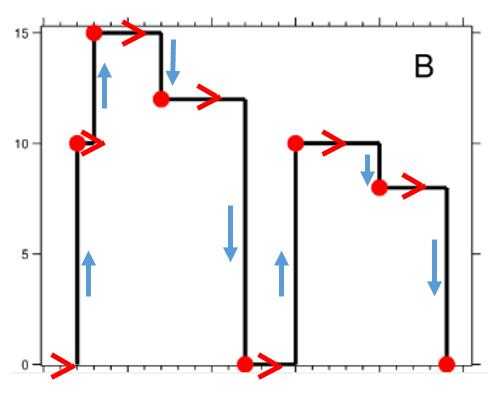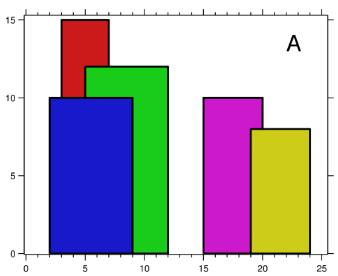# 题目描述（困难难度）# 思路分析？

https://www.geeksforgeeks.org/the-skyline-problem-using-divide-and-conquer-algorithm/

https://leetcode.com/problems/the-skyline-problem/discuss/61281/Java-divide-and-conquer-solution-beats-96# 解法一

Skyline1 = {(1, 11),  (3, 13),  (9, 0),  (12, 7),  (16, 0)}
Skyline2 = {(14, 3),  (19, 18), (22, 3), (23, 13),  (29, 0)}

Skyline1 存储第一组的解。
Skyline2 存储第二组的解。

Result 存储合并后的解, Result = {}

h1 表示将 Skyline1 中的某个关键点加入 Result 中时, 当前关键点的高度
h2 表示将 Skyline2 中的某个关键点加入 Result 中时, 当前关键点的高度

h1 = 0, h2 = 0
i = 0, j = 0

(1, 11),  (3, 13),  (9, 0),  (12, 7),  (16, 0)
^
i
(14, 3),  (19, 18), (22, 3), (23, 13),  (29, 0)
^
j

x 取 1, 接下来更新 height
h1 = 11, height = max(h1, h2) = max(11, 0) = 11

Result = {(1, 11)}
i 后移, i = i + 1 = 2

(1, 11),  (3, 13),  (9, 0),  (12, 7),  (16, 0)
^
i
(14, 3),  (19, 18), (22, 3), (23, 13),  (29, 0)
^
j

x 取 3, 接下来更新 height
h1 = 13, height = max(h1, h2) = max(13, 0) = 13

Result = {(1, 11), (3, 13)}
i 后移, i = i + 1 = 3

(9, 0) 和 (12, 7) 同理

Result 为 {(1, 11), (3, 13), (9, 0), (12, 7)}
i = 4

(1, 11),  (3, 13),  (9, 0),  (12, 7),  (16, 0)
^
i
(14, 3),  (19, 18), (22, 3), (23, 13),  (29, 0)
^
j

x 取 14, 接下来更新 height
h2 = 3, height = max(h1, h2) = max(7, 3) = 7

Result = {(1, 11), (3, 13), (9, 0), (12, 7), (14, 7)}
j 后移, j = j + 1 = 2

(1, 11),  (3, 13),  (9, 0),  (12, 7),  (16, 0)
^
i
(14, 3),  (19, 18), (22, 3), (23, 13),  (29, 0)
^
j

x 取 16, 接下来更新 height
h1 = 0, height = max(h1, h2) = max(0, 3) = 3

Result = {(1, 11), (3, 13), (9, 0), (12, 7), (14, 7), (16, 3)}
i 后移, i = i + 1 = 5

Result = {(1, 11), (3, 13), (9, 0), (12, 7), (14, 7), (16, 3),
(19, 18), (22, 3), (23, 13), (29, 0)}

Result = {(1, 11), (3, 13), (9, 0), (12, 7), (16, 3), (19, 18),
(22, 3), (23, 13), (29, 0)}


public List<List<Integer>> getSkyline(int[][] buildings) {
if(buildings.length == 0){
return  new ArrayList<>();
}
return merge(buildings, 0, buildings.length - 1);
}

private List<List<Integer>> merge(int[][] buildings, int start, int end) {

List<List<Integer>> res = new ArrayList<>();
//只有一个建筑, 将 [x, h], [y, 0] 加入结果
if (start == end) {
List<Integer> temp = new ArrayList<>();

temp = new ArrayList<>();
return res;
}
int mid = (start + end) >>> 1;
//第一组解
List<List<Integer>> Skyline1  = merge(buildings, start, mid);
//第二组解
List<List<Integer>> Skyline2  = merge(buildings, mid + 1, end);
//下边将两组解合并
int h1 = 0;
int h2 = 0;
int i = 0;
int j = 0;
while (i < Skyline1 .size() || j < Skyline2 .size()) {
long x1 = i < Skyline1 .size() ? Skyline1 .get(i).get(0) : Long.MAX_VALUE;
long x2 = j < Skyline2 .size() ? Skyline2 .get(j).get(0) : Long.MAX_VALUE;
long x = 0;
//比较两个坐标
if (x1 < x2) {
h1 = Skyline1 .get(i).get(1);
x = x1;
i++;
} else if (x1 > x2) {
h2 = Skyline2 .get(j).get(1);
x = x2;
j++;
} else {
h1 = Skyline1 .get(i).get(1);
h2 = Skyline2 .get(j).get(1);
x = x1;
i++;
j++;
}
//更新 height
int height = Math.max(h1, h2);
//重复的解不要加入
if (res.isEmpty() || height != res.get(res.size() - 1).get(1)) {
List<Integer> temp = new ArrayList<>();
}
}
return res;
}


long x1 = i < Skyline1 .size() ? Skyline1 .get(i).get(0) : Long.MAX_VALUE;
long x2 = j < Skyline2 .size() ? Skyline2 .get(j).get(0) : Long.MAX_VALUE;


Skyline1 或者 Skyline2 遍历完的时候，我们给他赋值为一个很大的数，这样的话我们可以在一个 while 循环中完成我们的算法，不用再单独考虑当一个遍历完的处理。

 if (res.isEmpty() || height != res.get(res.size() - 1).get(1)) {


# 解法二buildings  [2 9 10], [3 7 15], [5 12 12], [15 20 10], [19 24 8]

l(2,10) l(3,15) l(5,12) r(7,15) r(9,10) r(12,12)
l(15,10) l(19,8) r(20,10) r(24,8)
PriorityQueue = {0}, preMax = 0

l(2,10) 将 10 加入优先队列
preMax = 0, PriorityQueue  = {0 10}

l(3,15) 将 15 加入优先队列
preMax = 10, PriorityQueue  = {0 10 15}

l(5,12) 将 12 加入优先队列
preMax = 15, PriorityQueue  = {0 10 15 12}

res 不变

r(7,15) , 遇到右上角坐标, 将 15 从优先队列删除
preMax = 15, PriorityQueue  = {0 10 12}

r(9,10) , 遇到右上角坐标, 将 10 从优先队列删除
preMax = 12, PriorityQueue  = {0 12}

res 不变

r(12,12) , 遇到右上角坐标, 将 12 从优先队列删除
preMax = 12, PriorityQueue  = {0}



1. 当两个坐标都是左上角坐标，我们要将高度高的排在前边
2. 当两个坐标都是右上角坐标，我们要将高度低的排在前边
3. 当两个坐标一个是左上角坐标，一个是右上角坐标，我们需要将左上角坐标排在前边

public int compare(List<Integer> p1, List<Integer> p2) {
int x1 = p1.get(0);
int y1 = p1.get(1);
int x2 = p2.get(0);
int y2 = p2.get(1);
//不相等时候，按照 x 从小到大排序
if (x1 != x2) {
return x1 - x2;
//相等时候，只需要将高度相减就满足了上边的三条规则，可以尝试验证一下
} else {
return y1 - y2;
}
}


public List<List<Integer>> getSkyline(int[][] buildings) {
List<List<Integer>> points = new ArrayList<>();
List<List<Integer>> results = new ArrayList<>();
int n = buildings.length;
//求出左上角和右上角坐标, 左上角坐标的 y 存负数
for (int[] b : buildings) {
List<Integer> p1 = new ArrayList<>();

List<Integer> p2 = new ArrayList<>();
}
//将所有坐标排序
Collections.sort(points, new Comparator<List<Integer>>() {
@Override
public int compare(List<Integer> p1, List<Integer> p2) {
int x1 = p1.get(0);
int y1 = p1.get(1);
int x2 = p2.get(0);
int y2 = p2.get(1);
if (x1 != x2) {
return x1 - x2;
} else {
return y1 - y2;
}
}

});
//默认的优先队列是最小堆，我们需要最大堆，每次需要得到队列中最大的元素
Queue<Integer> queue = new PriorityQueue<>(new Comparator<Integer>() {
@Override
public int compare(Integer i1, Integer i2) {
return i2 - i1;
}
});
queue.offer(0);
int preMax = 0;

for (List<Integer> p : points) {
int x = p.get(0);
int y = p.get(1);
//左上角坐标
if (y < 0) {
queue.offer(-y);
//右上角坐标
} else {
queue.remove(y);
}
int curMax = queue.peek();
//最大值更新了, 将当前结果加入
if (curMax != preMax) {
List<Integer> temp = new ArrayList<>();
preMax = curMax;
}
}
return results;
}


TreeMap 的话 key 当然就是存高度了，因为可能添加重复的高度，所有value 的话存高度出现的次数即可。

public List<List<Integer>> getSkyline(int[][] buildings) {
List<List<Integer>> points = new ArrayList<>();
List<List<Integer>> results = new ArrayList<>();
int n = buildings.length;
//求出将左上角和右上角坐标, 左上角坐标的 y 存负数
for (int[] b : buildings) {
List<Integer> p1 = new ArrayList<>();

List<Integer> p2 = new ArrayList<>();
}
//将所有坐标排序
Collections.sort(points, new Comparator<List<Integer>>() {
@Override
public int compare(List<Integer> p1, List<Integer> p2) {
int x1 = p1.get(0);
int y1 = p1.get(1);
int x2 = p2.get(0);
int y2 = p2.get(1);
if (x1 != x2) {
return x1 - x2;
} else {
return y1 - y2;
}
}

});
TreeMap<Integer, Integer> treeMap = new TreeMap<>(new Comparator<Integer>() {
@Override
public int compare(Integer i1, Integer i2) {
return i2 - i1;
}
});
treeMap.put(0, 1);
int preMax = 0;

for (List<Integer> p : points) {
int x = p.get(0);
int y = p.get(1);
if (y < 0) {
Integer v = treeMap.get(-y);
if (v == null) {
treeMap.put(-y, 1);
} else {
treeMap.put(-y, v + 1);
}
} else {
Integer v = treeMap.get(y);
if (v == 1) {
treeMap.remove(y);
} else {
treeMap.put(y, v - 1);
}
}
int curMax = treeMap.firstKey();
if (curMax != preMax) {
List<Integer> temp = new ArrayList<>();
preMax = curMax;
}
}
return results;
}